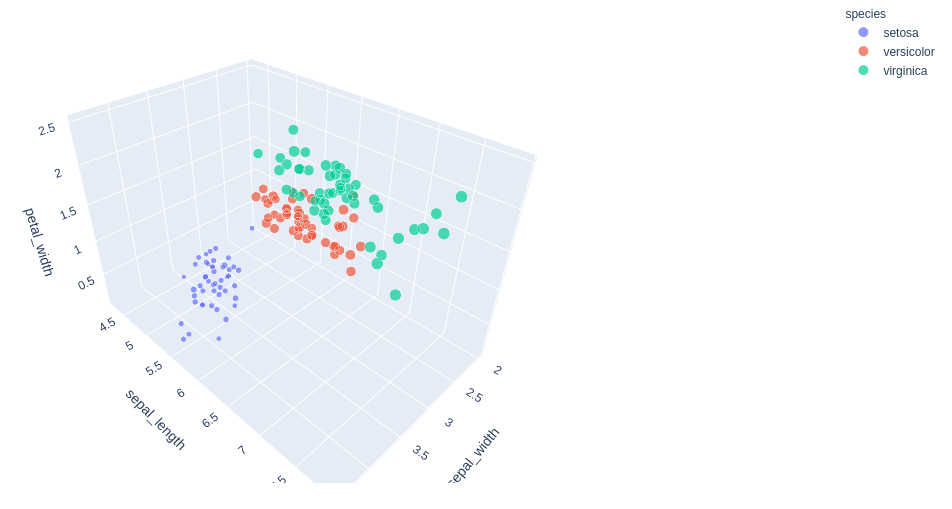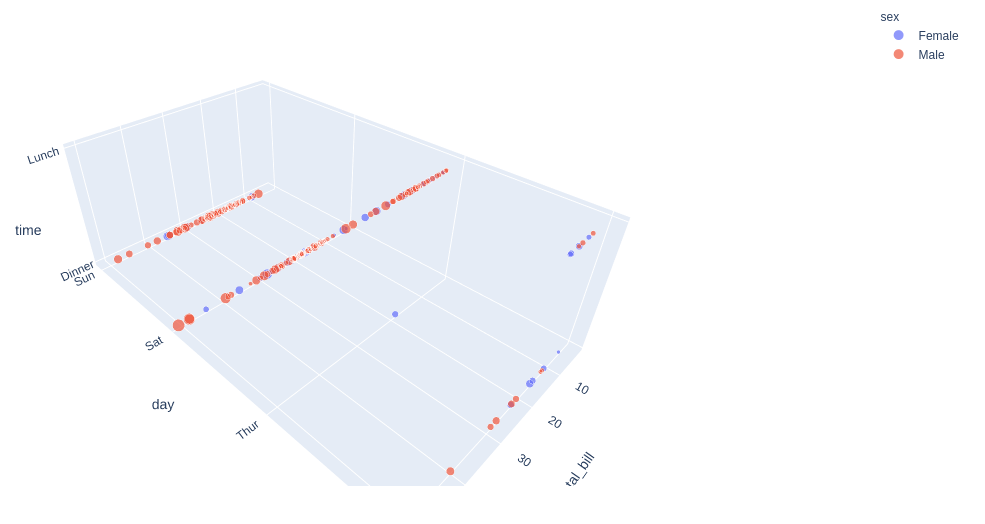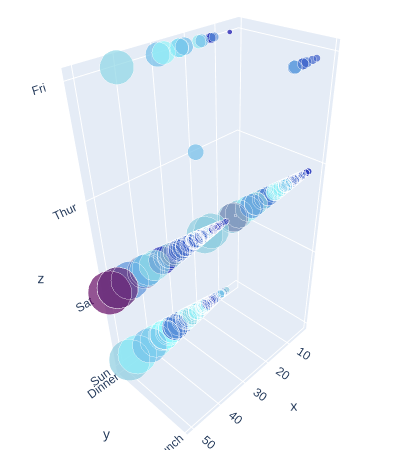# 3D Bubble chart using Plotly in Python

Plotly is a Python library that is used to design graphs, especially interactive graphs. It can plot various graphs and charts like histogram, barplot, boxplot, spreadplot, and many more. It is mainly used in data analysis as well as financial analysis. plotly is an interactive visualization library.

## 3D Bubble Chart in Plotly

A bubble chart can be created using the scatter_3d() method of plotly.express class. A bubble chart is a data visualization which helps to displays multiple circles (bubbles) in a 3d dimensional plot as same in 3d scatter plot. A bubble chart is primarily used to depict and show relationships between numeric variables.

Example 1: Using Iris dataset

## Python3

 `import` `plotly.express as px ` ` `  ` `  `df ``=` `px.data.iris() ` `fig ``=` `px.scatter_3d(df, x``=``'sepal_width'``, ` `                    ``y``=``'sepal_length'``,  ` `                    ``z``=``'petal_width'``, ` `                    ``size``=``'petal_length'``,  ` `                    ``color``=``'species'``) ` ` `  `fig.show()`

Output:Example 2: Using tips dataset

## Python3

 `import` `plotly.express as px ` ` `  ` `  `df ``=` `px.data.tips() ` `fig ``=` `px.scatter_3d(df, x``=``'total_bill'``, ` `                    ``y``=``'day'``, z``=``'time'``, ` `                    ``size``=``'tip'``, color``=``'sex'``) ` ` `  `fig.show()`

Output:## Customizing the Color bar

It can be edited using the color option of the Scatter3D() method of graph_objects class. Let’s look at the below example for a better understanding of the topic.

Example:

## Python3

 `import` `plotly.express as px ` `import` `plotly.graph_objects as go ` ` `  ` `  `df ``=` `px.data.tips() ` ` `  `fig ``=` `go.Figure(go.Scatter3d( ` `    ``x ``=` `df[``'total_bill'``], ` `    ``y ``=` `df[``'time'``], ` `    ``z ``=` `df[``'day'``], ` `    ``mode ``=` `'markers'``, ` `    ``marker ``=` `dict``( ` `        ``color ``=` `df[``'tip'``], ` `        ``size ``=` `df[``'total_bill'``], ` `        ``colorscale``=``[[``0``, ``'rgb(15, 10, 172)'``],  ` `                    ``[.``3``, ``'rgb(150, 255, 255)'``],  ` `                    ``[``1``, ``'rgb(100, 10, 100)'``]] ` `        ``) ` `)) ` ` `  `fig.show()`

Output:Whether you're preparing for your first job interview or aiming to upskill in this ever-evolving tech landscape, GeeksforGeeks Courses are your key to success. We provide top-quality content at affordable prices, all geared towards accelerating your growth in a time-bound manner. Join the millions we've already empowered, and we're here to do the same for you. Don't miss out - check it out now!

Previous
Next